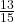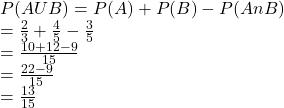## If P(A) = 2/3, P(B) = 4/5 and P(AnB) = 3/5, what is P(AuB)?

Question

If P(A) = 2/3, P(B) = 4/5 and P(AnB) = 3/5, what is P(AuB)?

in progress 0
5 months 2021-08-11T10:48:19+00:00 2 Answers 10 views 0Step-by-step explanation:

We know that,

P(AUB) = P(A) + P(B) – P(A n B)

So,hope this helps you.

Have a nice day!

13/15

Step-by-step explanation:

P(A∪B) = P(A) + P(B) – P(A∩B)

= 2/3 + 4/5 – 3/5

Getting a common denominator

2/3 *5/5 + 4/5*3/3  – 3/5 *3/3

10/15 + 12/15 – 9/15

13/15